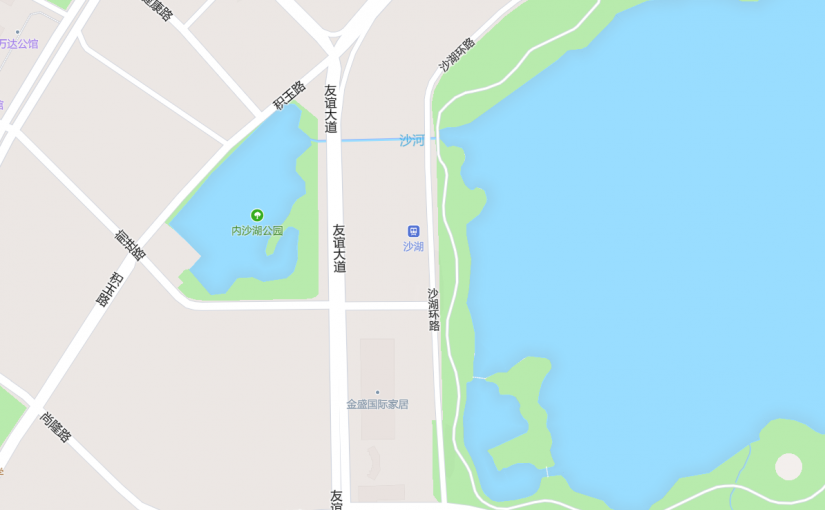# 多边形渐变色的实现

CSS3 的渐变效果在地图中的应用也是有的。比较常见的方式是，先将 CSS3 的径向渐变效果绘制在 canvas 上，再将 canvas 叠加到地图上。这样可以实现圆形的渐变效果，可以用于展示点的缓冲区范围。

## 相关概念

### Signed Distance Field（有向距离场）

```float sdPolygon( in vec2[N] v, in vec2 p )
{
float d = dot(p-v,p-v);
float s = 1.0;
for( int i=0, j=N-1; i<N; j=i, i++ )
{
vec2 e = v[j] - v[i];
vec2 w =    p - v[i];
vec2 b = w - e*clamp( dot(w,e)/dot(e,e), 0.0, 1.0 );
d = min( d, dot(b,b) );
bvec3 c = bvec3(p.y>=v[i].y,p.y<v[j].y,e.x*w.y>e.y*w.x);
if( all(c) || all(not(c)) ) s*=-1.0;
}
return s*sqrt(d);
}
```

## 后续优化

• 算法效率。这应该是阻挡这一效果在 Mapbox 中落地的很重要的一个因素吧。
• 现有库的稳定性，现有的 Javascript 版本的直骨架计算库都存在一些问题，从项目的 issue 中就可以看出来了。当然也可以尝试下将 cgal 编译为 wasm 来解决。
• 和矢量瓦片结合的问题，如何在数据被切分后，依旧保持效果的完整性？

## 发布者### Zhang

hope you enjoy my articles.

## 《多边形渐变色的实现》上有1条评论

1.匿名说道：

也可以在制作矢量瓦片的时候，就将 SDF 生成好，前端直接着色就行，这样就规避了算法效率的问题。